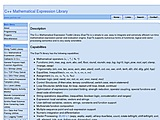### C++ Mathematical Expression Library 2.7182818284590452353602874713The C++ Mathematical Expression Toolkit Library (ExprTk) is a simple to use, easy to integrate and extremely efficient run-time mathematical expression parser and evaluation engine. ExprTk supports numerous forms of functional, logical and vector processing semantics and is very easily extendible.

Tags arithmetic expression math parser ast compile function trigonometry BSDL stable

#### Recent Releases

2.718281828459045235360287471329 Oct 2016 22:52 minor feature: